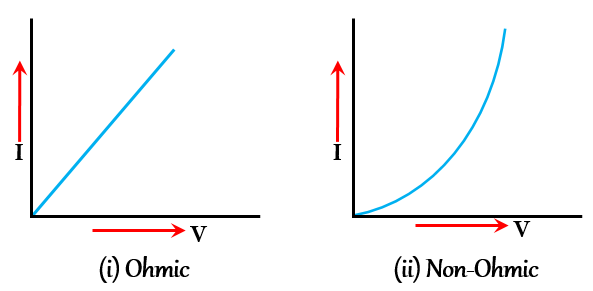# Define ohm's law and its limitations. Also, post graph of ohmic and non-ohmic materials.

Ohm's law states that the voltage across a resistor is directly proportional to the current flowing through it. Ohm's law is named for German physicist Georg Ohm.
In simple terms, Ohm's law gives the relationship between current, voltage, and resistance.
Mathematically this relationship is given as,
V = I ×  R, where
V = voltage across resistor
I = current
R = resistance

The limitations of Ohm's Law are as follow:-
1. Ohm's law is obeyed only when the temperature of the conductor remains constant.
2. It is valid for metal conductor only, not applicable for the non-metallic and semi-conductors conductors.

Non-Ohmic Materials - The materials which do not obey ohm’s law is known as non-ohmic materials. For example-  Semiconductors like Germanium and silicon do not obey Ohm’s law.
Ohmic materials - The materials which obey ohm’s law are known as ohmic material.
For example- All metals, like silver, gold, aluminium etc obey Ohm's law.

Graph of Ohmic and Non-ohmic materials are given below:-